# Creating and Inserting Basic Mathematical Expressions Without Prediction with Equatio

Just getting started with Equatio? Here are a few quick how-to’s in order to get started inserting math into your Google Docs without using Math Prediction. Please note: This is not an all inclusive list. You should also be sure to check out our video tutorials for Equatio or check out Inserting Geometry Symbols!

This article will cover inserting an exponent, creating a fraction or mixed number, square roots, and subscript, as well as listing some additional commands.

Below each article is an animated gif which shows the process in real time.

### Exponents and Subscript

To insert an exponent, use the caret (^) symbol to move your cursor up to the exponent slot, where you can then insert your exponent. Once you are finished, use the right arrow key (⇨) to move out of the exponent slot and continue typing your equation.

So let’s say we want to show the Pythagorean Theorem: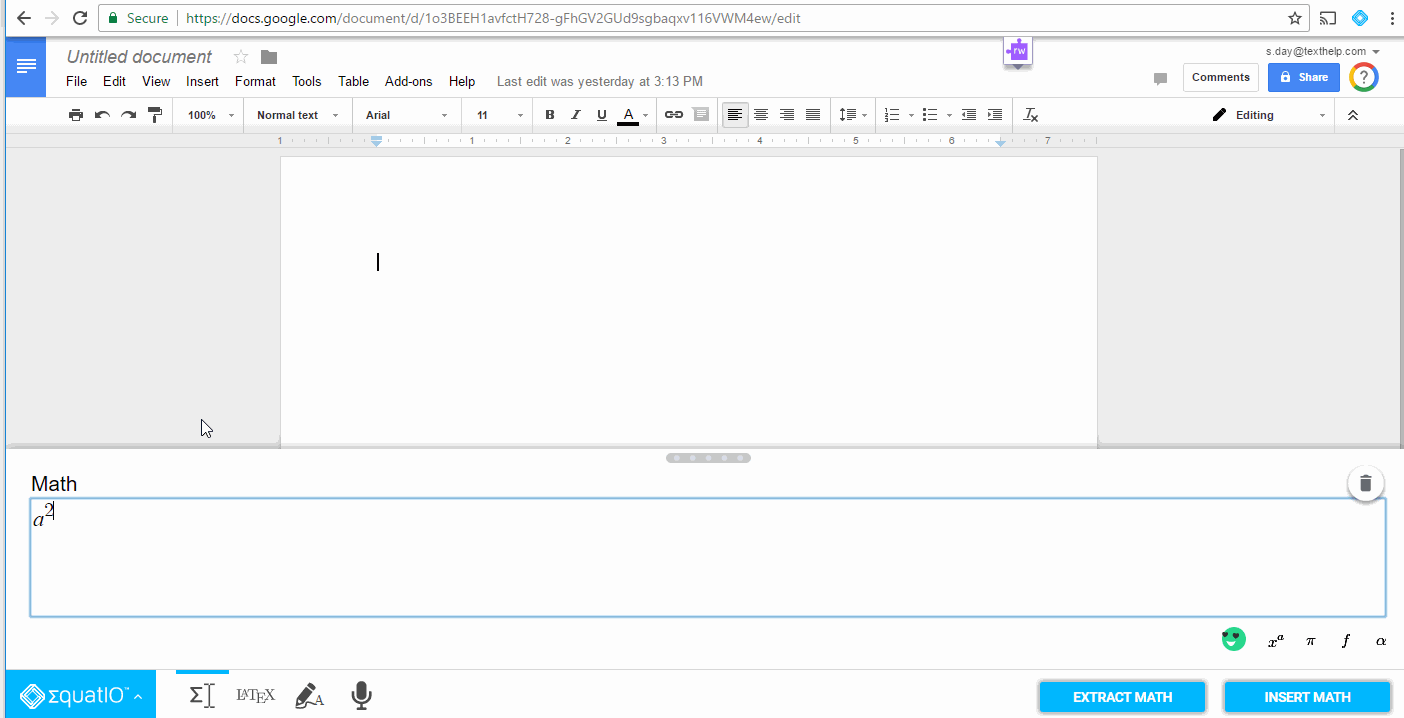For subscript, use the underscore (_) key to enter your subscript. Use your right arrow key (⇨) again when finished typing your subscript, to finish the rest of your equation.

So for example we can write out the chemical formula of a water molecule: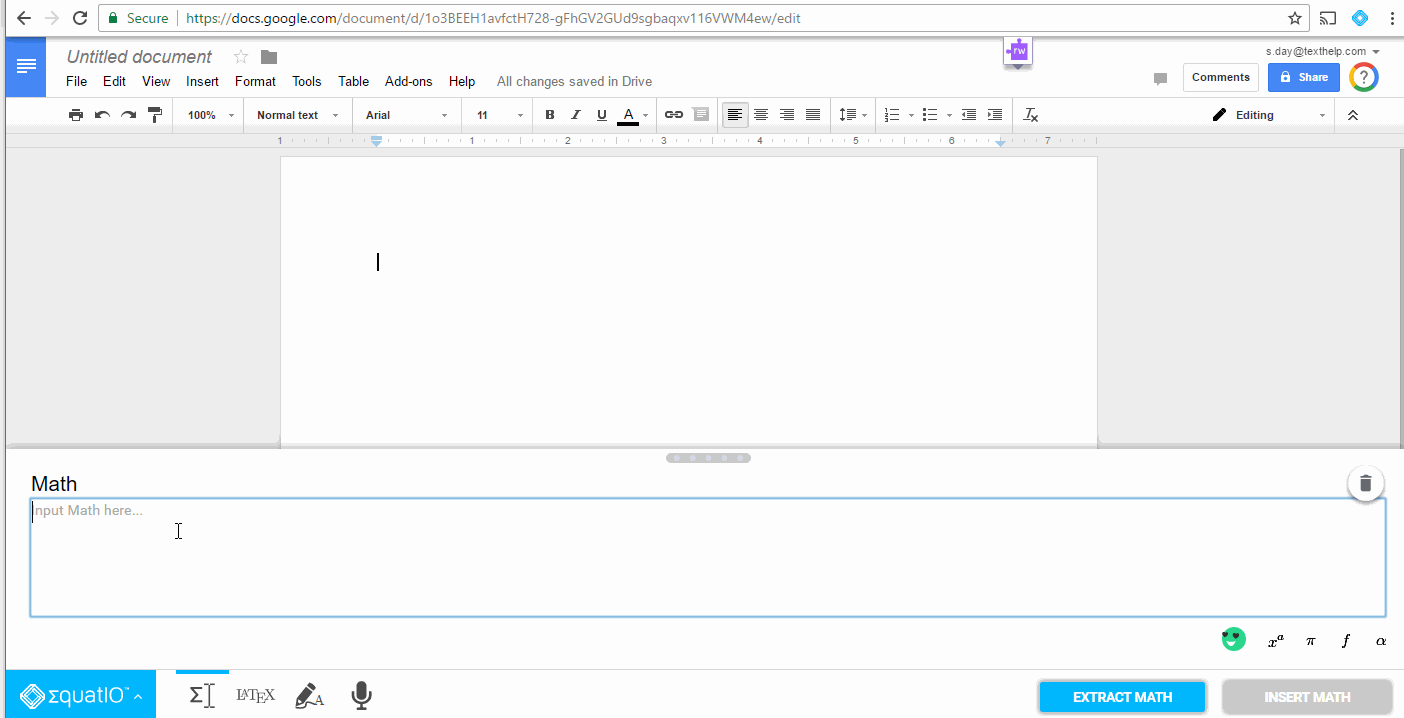### Fractions and Mixed Numbers

To insert a simple fraction, just type the numerator, press the / key, then type the denominator. Use the right arrow key (⇨) to continue typing the rest of your equation.

So if we wanted to add several fractions together, it would look like this: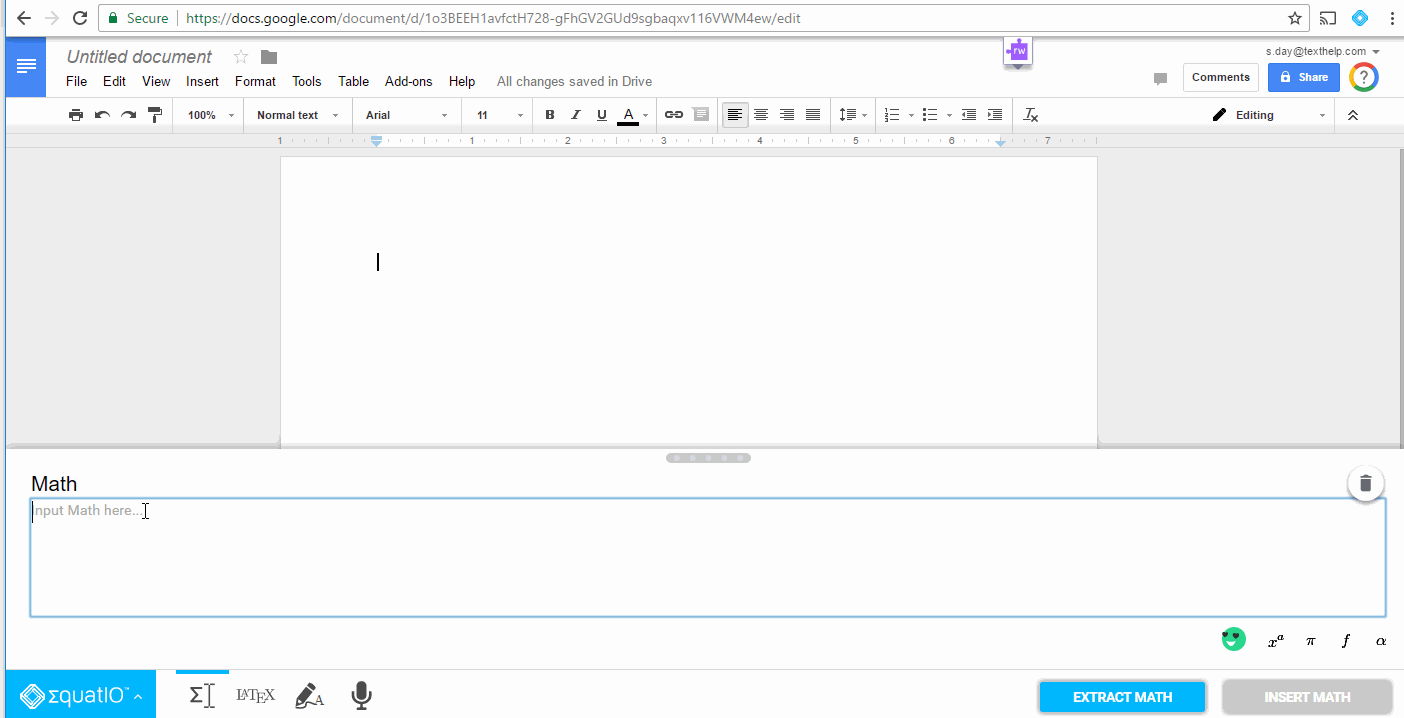We can also add a bit more complexity to these fractions by adding multiple terms to the numerator or denominator.

To do this, just type your numerator, highlight or select it, and then press the / key to insert the fraction.

When you’re done typing the terms in your denominator, use the right arrow key (⇨) to move on to the rest of your equation.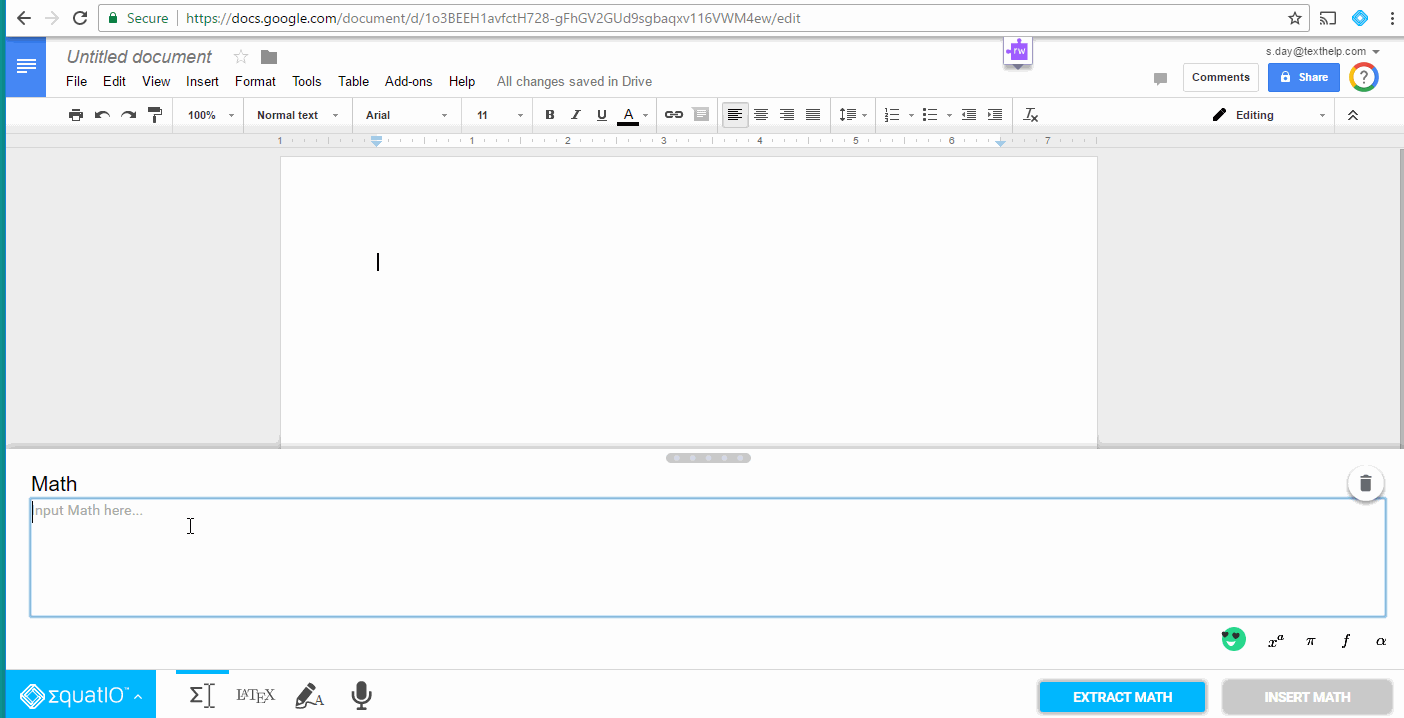### Square root

To insert a square root, just type \sqrt and then the Enter or Tab key to insert the square root symbol. Then just type the number or expression you want to include under the square root. Use the Enter key when finished to continue typing the rest of your equation.

So for example if we want to write the square root of 16, it would look like this: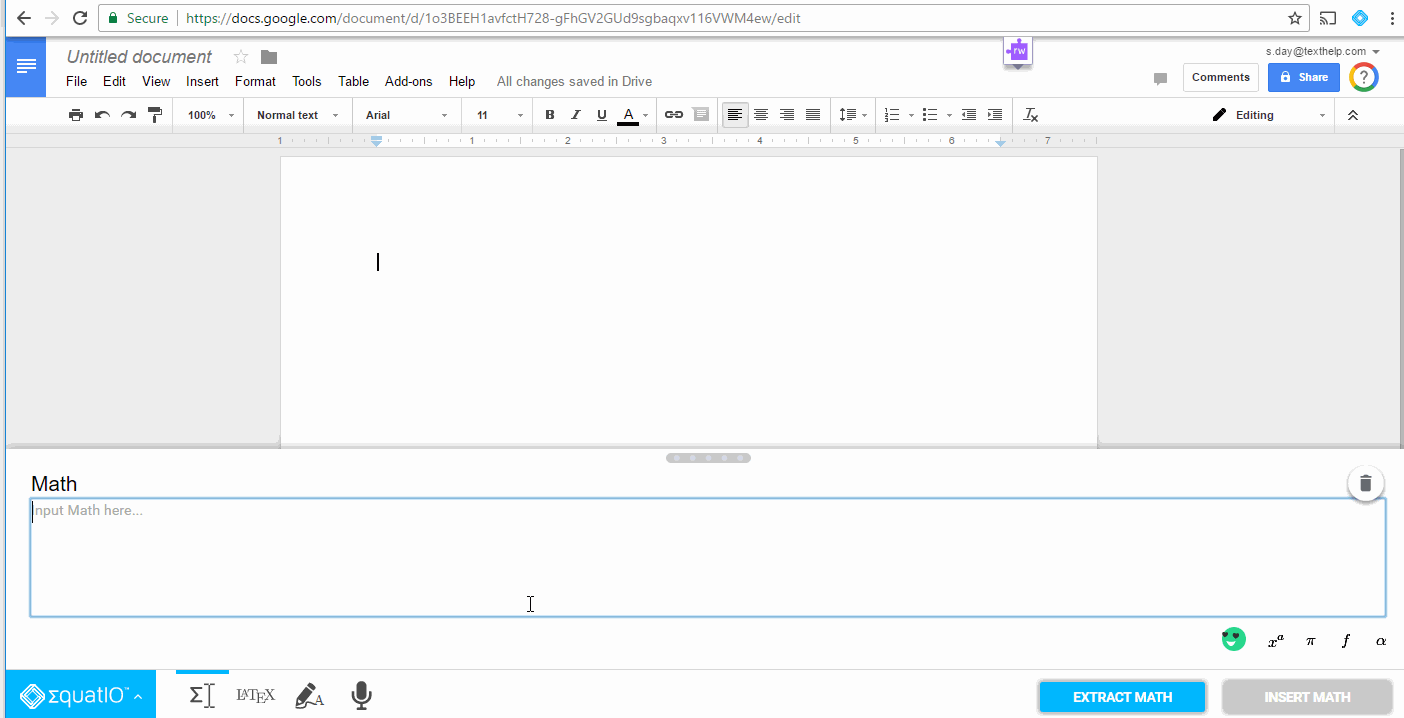\div = division sign

\times = multiplication sign

\cdot = dot multiplication sign

\pi = pi symbol

Greek symbols

\sqrt = cube root

\sqrt[n] = nth root